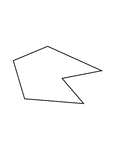Irregular Concave Hexagon

Illustration of an irregular hexagon. This is also an example of a concave polygon.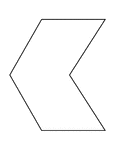Irregular Concave Hexagon

Illustration of an irregular hexagon. This is also an example of a concave polygon with symmetry.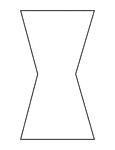Irregular Concave Hexagon

Illustration of an irregular hexagon. This is also an example of a concave polygon with symmetry.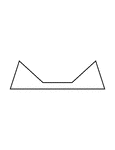Irregular Concave Hexagon

Illustration of an irregular hexagon. This is also an example of a concave polygon with symmetry.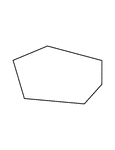Irregular Convex Hexagon

Illustration of an irregular convex hexagon.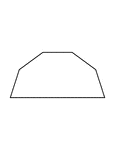Irregular Convex Hexagon

Illustration of an irregular convex hexagon with symmetry.